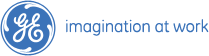# Placement Papers - GEGE PAPER - 20 FEB 2008
Posted by :
Sruthi
(241)
Hi Friends

1. If 2 boxes are selected at random, what is the probability that 2 boxes at side by side?

2. if a number "P457102Q" is divided by 18 then P&Q are?

3. 1050,510,216,106,24,16,5(find out error number)?

4. 1,3,5,11,21,....(fill this series)?

5. if G, S, N are done the work in 34,50,74 days. if they are combined to do the work G left 8 days before and S left 4days before then how many days N works?

6. box contains 700/-. box with equal 0.5, 1, 2/- coins then how many one rupee coins in box?

7. x+y+z=0, then X^2/yz + y^2/xz +z^2/xy=?

8. one circle is inscribbed in a square with area "9pi" then area of square ?

9. In a book SPAIN represented by 17598 and NARESH with 052314 then ASIN with?

10. How many triangles are there in given fig?